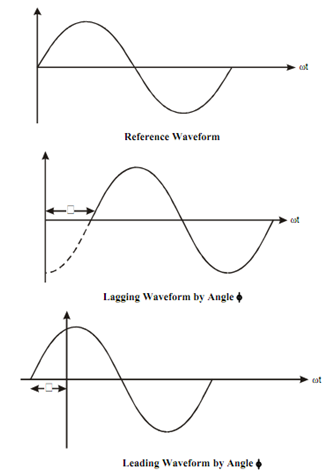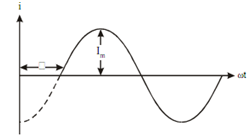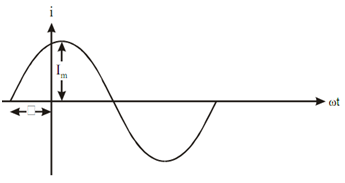## Phase Angle and Phase Difference Assignment Help

Assignment Help: >> Basic Definitions about the Alternating Quantity - Phase Angle and Phase Difference

Phase Angle:

This is the angle of alternating quantity with respect to the reference position.

Phase Difference:

While the maxima and minima of two sinusoidal alternating quantities (of same frequency) do not occur at the same instant of time, then these two quantities are said to have phase difference.Figure

If the current waveform of Figure (a) is supposed to be reference wave, then Figure (a) illustrated the lagging current.Figure (a) : Reference Current Waveform

i = I m  sin (ωt - φ)

This equation illustrated that current is lagging (or running behind) the reference wave by an angle φ.

Leading Current by an Angle φ

Figure (b) illustrates the leading current by an angle φ by the reference wave of Figure (a).

i = I m  sin (ωt + φ)Figure (b) : Leading Current Waveform#### Assured A++ Grade

Get guaranteed satisfaction & time on delivery in every assignment order you paid with us! We ensure premium quality solution document along with free turntin report!

All rights reserved! Copyrights ©2019-2020 ExpertsMind IT Educational Pvt Ltd